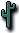##C source code for Base 64

Here's the C source code for the Base 64 encoder/decoder.

 `File:` `base64.c` `Created:` `Saturday, April 5, 1997; 1:30:13 PM` `Modified: ` `Tuesday, April 8, 1997; 7:52:28 AM`

```/*
Dave Winer, dwiner@well.com, UserLand Software, 4/7/97

I built this project using Symantec C++ 7.0.4 on a Mac 9500.

We needed a handle-based Base 64 encoder/decoder. Looked around the
net, found a bunch of code that couldn't easily be adapted to
in-memory stuff. Most of them work on files to conserve memory. This
is inelegant in scripting environments such as Frontier.

Anyway, so I wrote an encoder/decoder. Docs are being maintained
on the web, and updates at:

http://www.scripting.com/midas/base64/

If you port this code to another platform please put the result up
on a website, and send me a pointer. Also send email if you think this
isn't a compatible implementation of Base 64 encoding.

BTW, I made it easy to port -- layering out the handle access routines.
Of course there's a small performance penalty for this, and if you don't
like it, change it. Thanks!
*/

#include <appletdefs.h>
#include <iac.h>
#include "base64.h"

static char encodingTable  = {

'A','B','C','D','E','F','G','H','I','J','K','L','M','N','O','P',

'Q','R','S','T','U','V','W','X','Y','Z','a','b','c','d','e','f',

'g','h','i','j','k','l','m','n','o','p','q','r','s','t','u','v',

'w','x','y','z','0','1','2','3','4','5','6','7','8','9','+','/'
};

static unsigned long gethandlesize (Handle h) {

return (GetHandleSize (h));
} /*gethandlesize*/

static boolean sethandlesize (Handle h, unsigned long newsize) {

SetHandleSize (h, newsize);

return (MemError () == noErr);
} /*sethandlesize*/

static unsigned char gethandlechar (Handle h, unsigned long ix) {

return ((*h) [ix]);
} /*gethandlechar*/

static void sethandlechar (Handle h, unsigned long ix, unsigned char ch) {

(*h) [ix] = ch;
} /*sethandlechar*/

static boolean encodeHandle (Handle htext, Handle h64, short linelength) {

/*
encode the handle. some funny stuff about linelength -- it only makes
sense to make it a multiple of 4. if it's not a multiple of 4, we make it
so (by only checking it every 4 characters.

further, if it's 0, we don't add any line breaks at all.
*/

unsigned long ixtext;
unsigned long lentext;
unsigned long origsize;
long ctremaining;
unsigned char ch;
unsigned char inbuf , outbuf ;
short i;
short charsonline = 0, ctcopy;

ixtext = 0;

lentext = gethandlesize (htext);

while (true) {

ctremaining = lentext - ixtext;

if (ctremaining <= 0)
break;

for (i = 0; i < 3; i++) {

unsigned long ix = ixtext + i;

if (ix < lentext)
inbuf [i] = gethandlechar (htext, ix);
else
inbuf [i] = 0;
} /*for*/

outbuf  = (inbuf  & 0xFC) >> 2;

outbuf  = ((inbuf  & 0x03) << 4) | ((inbuf  & 0xF0) >> 4);

outbuf  = ((inbuf  & 0x0F) << 2) | ((inbuf  & 0xC0) >> 6);

outbuf  = inbuf  & 0x3F;

origsize = gethandlesize (h64);

if (!sethandlesize (h64, origsize + 4))
return (false);

ctcopy = 4;

switch (ctremaining) {

case 1:
ctcopy = 2;

break;

case 2:
ctcopy = 3;

break;
} /*switch*/

for (i = 0; i < ctcopy; i++)
sethandlechar (h64, origsize + i, encodingTable [outbuf [i]]);

for (i = ctcopy; i < 4; i++)
sethandlechar (h64, origsize + i, '=');

ixtext += 3;

charsonline += 4;

if (linelength > 0) { /*DW 4/8/97 -- 0 means no line breaks*/

if (charsonline >= linelength) {

charsonline = 0;

origsize = gethandlesize (h64);

if (!sethandlesize (h64, origsize + 1))
return (false);

sethandlechar (h64, origsize, '\n');
}
}
} /*while*/

return (true);
} /*encodeHandle*/

static boolean decodeHandle (Handle h64, Handle htext) {

unsigned long ixtext;
unsigned long lentext;
unsigned long origsize;
unsigned long ctremaining;
unsigned char ch;
unsigned char inbuf , outbuf ;
short i, ixinbuf;
boolean flignore;
boolean flendtext = false;

ixtext = 0;

lentext = gethandlesize (h64);

ixinbuf = 0;

while (true) {

if (ixtext >= lentext)
break;

ch = gethandlechar (h64, ixtext++);

flignore = false;

if ((ch >= 'A') && (ch <= 'Z'))
ch = ch - 'A';

else if ((ch >= 'a') && (ch <= 'z'))
ch = ch - 'a' + 26;

else if ((ch >= '0') && (ch <= '9'))
ch = ch - '0' + 52;

else if (ch == '+')
ch = 62;

else if (ch == '=') /*no op -- can't ignore this one*/
flendtext = true;

else if (ch == '/')
ch = 63;

else
flignore = true;

if (!flignore) {

short ctcharsinbuf = 3;
boolean flbreak = false;

if (flendtext) {

if (ixinbuf == 0)
break;

if ((ixinbuf == 1) || (ixinbuf == 2))
ctcharsinbuf = 1;
else
ctcharsinbuf = 2;

ixinbuf = 3;

flbreak = true;
}

inbuf [ixinbuf++] = ch;

if (ixinbuf == 4) {

ixinbuf = 0;

outbuf  = (inbuf  << 2) | ((inbuf  & 0x30) >> 4);

outbuf  = ((inbuf  & 0x0F) << 4) | ((inbuf  & 0x3C) >> 2);

outbuf  = ((inbuf  & 0x03) << 6) | (inbuf  & 0x3F);

origsize = gethandlesize (htext);

if (!sethandlesize (htext, origsize + ctcharsinbuf))
return (false);

for (i = 0; i < ctcharsinbuf; i++)
sethandlechar (htext, origsize + i, outbuf [i]);
}

if (flbreak)
break;
}
} /*while*/

exit:

return (true);
} /*decodeHandle*/

void base64encodeVerb (void) {

Handle h64, htext;
short linelength;

if (!IACgettextparam ((OSType) keyDirectObject, &htext))
return;

if (!IACgetshortparam ((OSType) 'line', &linelength))
return;

h64 = NewHandle (0);

if (!encodeHandle (htext, h64, linelength))
goto error;

DisposHandle (htext);

IACreturntext (h64);

return;

error:

IACreturnerror (1, "\perror encoding the Base 64 text");
} /*base64encodeVerb*/

void base64decodeVerb (void) {

Handle h64, htext;

if (!IACgettextparam ((OSType) keyDirectObject, &h64))
return;

htext = NewHandle (0);

if (!decodeHandle (h64, htext))
goto error;

DisposHandle (h64);

IACreturntext (htext);

return;

error:

IACreturnerror (1, "\perror decoding the Base 64 text");
} /*base64decodeVerb*/

```